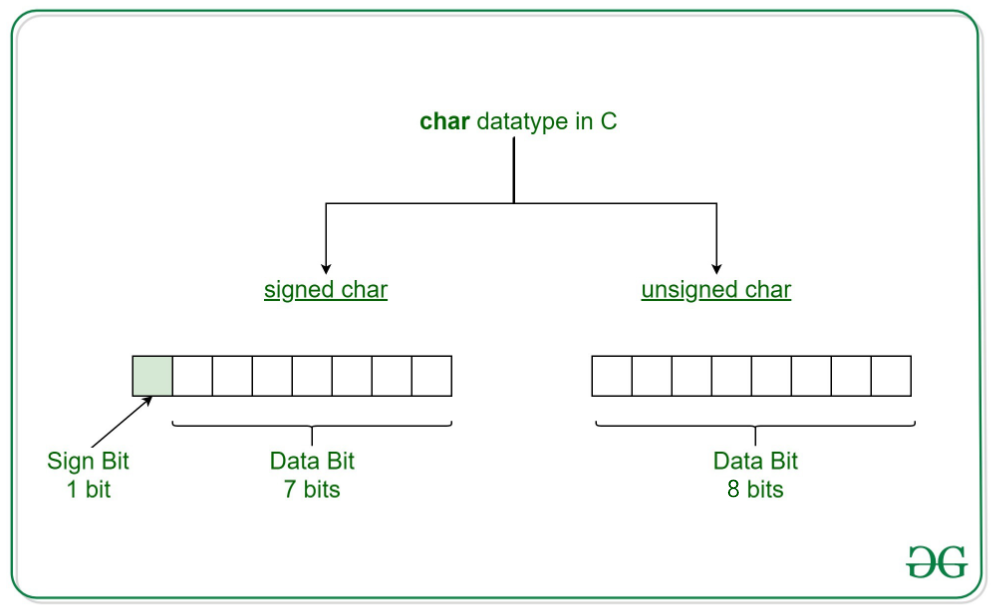# unsigned char in C with Examples

• Difficulty Level : Easy
• Last Updated : 06 Aug, 2020

char is the most basic data type in C. It stores a single character and requires a single byte of memory in almost all compilers.

Now character datatype can be divided into 2 types:

1. signed char
2. unsigned charunsigned char is a character datatype where the variable consumes all the 8 bits of the memory and there is no sign bit (which is there in signed char). So it means that the range of unsigned char data type ranges from 0 to 255.

Syntax:

```unsigned char [variable_name] = [value]
```

Example:

```unsigned char ch = 'a';
```
• Initializing an unsigned char: Here we try to insert a char in the unsigned char variable with the help of ASCII value. So the ASCII value 97 will be converted to a character value, i.e. ‘a’ and it will be inserted in unsigned char.

 `// C program to show unsigned char`` ` `#include `` ` `int` `main()``{`` ` `    ``int` `chr = 97;``    ``unsigned ``char` `i = chr;``    ``printf``(``"unsigned char: %c\n"``, i);`` ` `    ``return` `0;``}`

Output:

```unsigned char: a
```

Initializing an unsigned char with signed value: Here we try to insert a char in the unsigned char variable with the help of ASCII value. So the ASCII value -1 will be first converted to a range 0-255 by rounding. So it will be 255. Now, this value will be converted to a character value, i.e. ‘ÿ’ and it will be inserted in unsigned char.

 `// C program to show unsigned char`` ` `#include `` ` `int` `main()``{`` ` `    ``int` `chr = -1;``    ``unsigned ``char` `i = chr;``    ``printf``(``"unsigned char: %c\n"``, i);`` ` `    ``return` `0;``}`

Output:

```unsigned char: ÿ
```

My Personal Notes arrow_drop_up